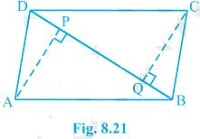# ABCD is a parallelogram and AP and CQ are perpendiculars from vertices A and C on diagonal BD (see Fig.) . Show that (i) ΔAPB ≅ ΔCQD (ii) AP = CQSolution

ABCD is a parallelogram

DP = BQ

(i) ∆APB ≅ ∆CQD

(i) In ∆APB and ∆CQD, we have

∠APB = ∠CQD [Each 90°]

AB = CD [ Opposite sides of a parallelogram ABCD are equal]

∠ABP = ∠CDQ [Alternate angles are equal as AB || CD and BD is a transversal]

Hence, ∆APB  ≅  ∆CQD [By AAS congruency]

(ii) AP = CQ

As, ∆APB ≅ ∆CQD [Proved]

AP = CQ [By C.P.C.T.]